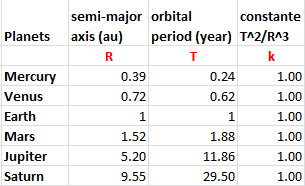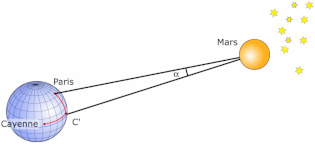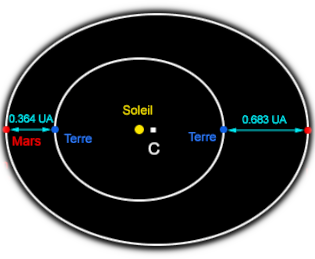# First measurement of the Earth-Sun distance

## Relative size of the solar systemAutomatic translation Category: Planets and dwarf planets
Updated October 22, 2021

At the beginning of the 17th century, scientists had no idea of the dimensions of the solar system. However, thanks to the laws of Johannes Kepler (1571−1630) we know the relative distances of the five planets of the time compared to the Sun. In other words, we know the distances in an unknown unit, the Earth-Sun distance, but we don't know the Earth-Sun distance in km.
Johannes Kepler's 3rd law, formulated in 1618, shows the relationship between the semi-major axis of a planet's orbit and its period of rotation around the Sun (table opposite). The cube of the semi-major axis is equal to the square of the period of rotation (T2 / R3 = k) which positions the orbit of Mercury at 0.39 AU (astronomical unit created much later in 1958) and that of Saturn at 9.55 astronomical units from the Sun.
The measurement of a single distance (planet-Sun) will give the scale of the entire system and therefore the Earth-Sun distance in km as expected by astronomers.

nota : Kepler's laws
Kepler's first law "law of orbits" (published in 1609 in Astronomia nova), stipulates that all bodies in orbit describe elliptical trajectories, of which the Sun occupies one of the foci.
Kepler's second law "law of areas" (published in 1609 in Astronomia nova), states that equal areas are scanned in equal times.
Kepler's third law "the law of periods" (published in 1619 in Harmonices Mundi), states that the square of the sidereal period of a planet (time between two successive passages in front of a star) is directly proportional to the cube of the half major axis of the elliptical trajectory of the planet.

In 1687, Isaac Newton discovered the law of gravitation which allowed him to explain Kepler's three laws.## Earth-Mars distance measurement

As paradoxical as it may seem, it is by measuring the Earth-Mars distance that 17th century astronomers and mathematicians were able to get an idea of the dimensions of the solar system.
At that time, observation was mainly summarized by measuring the angles of celestial objects seen from the Earth. Then it is the calculations of trigonometry which give the distances.
How was the Earth-Mars distance calculated for the first time?
In September 1672, Jean-Dominique Cassini (1625−1712), Jean Picard (1620−1682) and Jean Richer (1630−1696) measure the horizontal parallax of Mars when this planet passed closest to the Earth (Mars is then, seen from the Earth, opposite the Sun). To carry out this measurement, it is necessary to observe the positions of Mars in relation to the stars much more distant from two very distant points.
Cassini from Paris and Richer from Cayenne measures the parallax of Mars. This measurement related to the base formed by the equatorial radius of the Earth gives a horizontal parallax of p = 24 "⇒ Earth-Mars = 54 746 000 km.
To determine the parallax of Mars from observations made from Paris and Cayenne, it is necessary to know with maximum precision the difference between the latitudes and the difference between the longitudes of Paris and Cayenne.

Latitude (see nota) is easy to measure but longitude (see nota) is much more difficult at the time.
Cassini specifies however that he measured the longitude by several methods to obtain an average:
- The observation of an eclipse whose various phases are identified in hours of the meridian of Paris, in the astronomical collections.
- The observation of eclipses of the Galilean satellites of Jupiter which occur several times a day.
- The observation of the meridian heights of the Sun.
The whole gives an average of 3 h 39 min ± 10 min.
Richer and Cassini therefore observe the planet Mars during the month of September 1672. Mars will pass near an Aquarius star (Ψ Aquarii). In the image opposite, point C corresponds to the latitude of C (Cayenne) and the longitude of P (Paris). Cassini uses two simultaneous observations of Mars, one from P, the other from C'. The Paris Cayenne angle from Mars is the angle α = 14 ". But the parallax of Mars is the angle at which Mars sees the radius of the Earth. The calculation of proportionality between the Paris Cayenne angle and the parallax of Mars gives 24 "± 5"
Once the parallax of Mars is known, trigonometry makes it possible to obtain the distance Earth-Mars (D) according to the radius (R) of the Earth:
D = R×3600/24×180/π i.e. 8600 R
D = 8600 * 6371 = 54 790 600 km### Image: Cassini measurements made it possible to measure the angle α. Two observers are placed at two sufficiently distant points on the Earth (Paris and Cayenne for example) and note the configuration of the stars surrounding the observed star. They can thus measure the angles from the observation points then deduce the parallax α (in radians) then the Earth-Mars distance.

nota: Latitude is the north or south position of a point on Earth, in other words it is the angle with the equatorial plane (from 0 ° at the equator to 90 ° at the north pole and -90 ° at the south pole).

nota: Longitude is the East-West position of a point on Earth, which corresponds to a time difference with the plane of the Greenwich meridian (prime meridian, 0 ° longitude). This shift varies from + 180 ° west to -180 ° east).

## Calculation of the Earth-Sun distance

What is the Earth-Sun distance?
As soon as the Earth-Mars distance was known, the Earth-Sun distance could be calculated using Kepler's third law.
Cassini knew that Mars was at 0.38 AU = 3/8 AU. Earth-Sun = 8/3 x 8600 R = 23000 R ± 5000 terrestrial rays.
Cassini gives us the value of the terrestrial radius in leagues: R = 1500 leagues.
At the time there were a large number of definitions of the league depending on the region.
Let us suppose that the terrestrial radius is given in league of the abbot Picard which from 1671 is equal to 3707 meters.
R = 1500 x 3,707 = 5560.5 km from where:
Earth-Sun = 127,891,500 km at ± 27,802,500 km
This remarkable result constitutes the first measurement of the Earth-Sun distance.
Thanks to Kepler's third law, this distance will give the scale of the entire solar system T2/R3=k (table above).

### Image: calculation of distances thanks to the orbits of the Earth and Mars C = center of the ellipse representing the orbit of Mars Semi-major axis of the orbit of Mars = C → Mars Eccentricity of the orbit of Mars = CS / CM = 0.093 CM = CS + ST + TM CM = TM + ST / 1-CS TM = 54,790,600 km PT (Earth period) = 1 year (365 days) PM (Mars period) = 1.88 an (686 days) ⇒ TS = TM / 1-CS(PM/PT)2/3 -1 1-CS(PM/PT)2/3 -1 = 0.38 soit 3/8 ⇒ TS = 54,790,600 x 8/3 = 150,000,000 km1997 © Astronoo.com − Astronomy, Astrophysics, Evolution and Earth science. Contact    Mentions légales